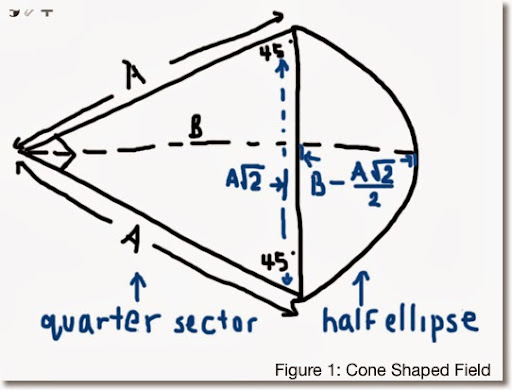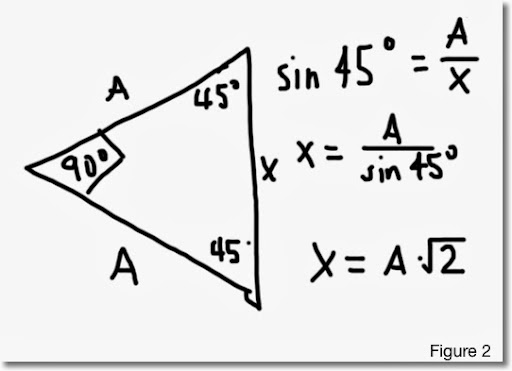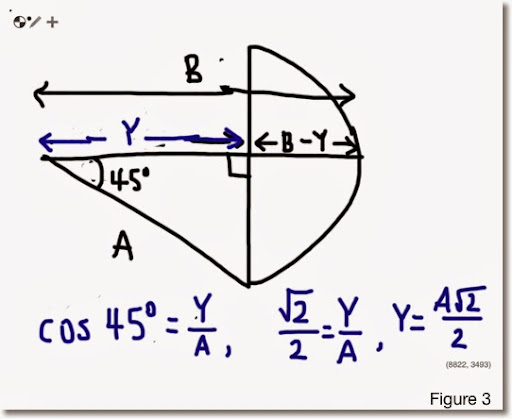## Friday, January 10, 2014

### Geometry: Area of a Cone-Shaped Field

Honestly the name of the object presented on this blog entry is made up names because I don't think there is an official geometrical name. This was inspired by trying to find areas of baseball fields. Hopefully this provides inspiration and maybe insight.

Area of a Cone-Shaped Field

Description: A triangle with line segments splitting away from each other at an 90° angle. After a certain distance, where the triangle stops, and half-ellipse is attached at the end.Measurements given:
A = length of a vector
B = length of a segment from the vertex to the outer point of the half ellipse. This line divides the shape in symmetric halves on each side.

The area of the cone-shaped field can be found in two parts:
(I) Area of the triangle
(II) Area of the half-ellipse
Total Area = (I) + (II)

Part (I):Let X be the length of the line connecting the end point of the out reaching lines. (See Figure 2)

Then from trigonometry, sin 45° = A/X which implies that X = A*√2. This will come in handy for part (II).

Taking advantage of the fact that the triangle is a right triangle, we can use the basic area formula 1/2 * base * height and the area of (I) is:

1/2 * A^2.

(II) Finding the area of the half ellipse. Let's start with the general formula
π * length of the semi-major axis * length of the semi-minor axis.

Since we are working with half of the ellipse, the general formula becomes
1/2 * π * length of the semi-major axis * length of the semi-minor axis.

I am not worried whether which of the axis is the major and which one is the minor. I am going for a general formula.

From Figure 2 above, X = A*√2. This is one of the axis. Half of this length is 1/2*A*√2.

Take a look at Figure 3 below to see how we will obtain the length of the other axis:Break the length B into two parts. Let Y be the length of the line segment from the vertex to the end of the triangle. For finding the area of the half ellipse, we want the quantity B - Y, which represents the length of one of the semi-axis.

The line bisects the 90° angle into two 45° angles. Using trigonometry, Y = A*√2/2.

Hence, our semi-axis length is B - Y = B - A*√A.

Then the area of the half-ellipse is:

1/2 * π * (A*√2/2) * (B - A*√2/2)
= π/4 * (A*B*√2 - A^2)

Having the two parts completed, the total area of the cone-shaped field is:

(I) + (II) = A^2/2 + π/4 * (A * B * √2 - A^2)

The take away point is that if you have an unusual shape and if you can recognize at least parts of familiar shapes, you can come up with a fairly closed formula for calculating the area for that shape.

Until then, have a great day and get creative!

Eddie

This blog is property of Edward Shore. 2014

#### 2 comments:

1.90minup ข่าวกีฬา ฟุตบอล ผลบอล วิเคราะห์บอล พรีเมียร์ลีก ฟุตบอลไทย
ข่าวกีฬา
ข่าวฟุตบอล
ฟุตบอลไทย
ฟุตบอล
วิเคราะห์บอล
ผลบอล
90minup

2.I think its must be helpful and informative for us. Thanks for sharing your nice post.ยูฟ่าเบท

### Swiss Micros DM16L: Advanced Boolean and Factorial (up to 20)

Swiss Micros DM16L:   Advanced Boolean and Factorial (up to 20) Introduction The program listing, for the Swiss Micros DM16L and Hewlett Pac...